Digital & Computing

### Become an OU studentDigital communications

Start this free course now. Just create an account and sign in. Enrol and complete the course for a free statement of participation or digital badge if available.

# 1.2 Wavelength or frequency?

In this unit you will find frequent references both to the wavelength of light and the frequency of light. It is important to be able to work with both, and convert from one to the other. In the context of optical-fibre communication, the light radiation we are concerned with is usually in the infrared region of the spectrum.

To identify the position in the electromagnetic spectrum it is usual to refer to the wavelength of light, rather than its frequency. Thus I will refer to the ‘1300 nm window’ rather than the 2﻿.﻿3 × 10﻿14 Hz (230 THz) window. T is the SI prefix ‘tera’, the multiplier for 1012.

When talking about the details of the optical spectrum both wavelengths and frequencies are used. In dense wavelength division multiplexing, for example, the carriers are often referred to by their frequencies rather than their wavelengths, and it is important to be able to convert readily from one to the other.

The conversion between wavelength (lambda, λ) and frequency (f) uses the formula:where c is the speed of light in free space (i.e. in a vacuum) which is

3.00 × 108 m s−1 to three significant figures. This may be rearranged to give: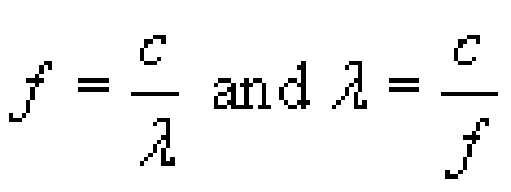Note that because the speed used is that of light in free space, references to wavelength are to the wavelength of the light in free space, which is different from (i.e. longer than) the wavelength of the same light in a fibre. When light enters a fibre from free space (or air) it slows down but the frequency remains the same, so the wavelength decreases.

You need to be careful when converting between wavelength and frequency spacings or ranges, rather than individual values. For example, let's suppose you know the output from a light-emitting diode (LED) contains wavelengths between 1300 nm and 1320 nm so that the spectral width is 20 nm and you want to know the frequency range in hertz.

To get the exact frequency range you need to convert each end of the range to frequencies and subtract one from the other. Thus the spectrum of the LED output in this example extends from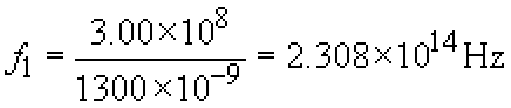to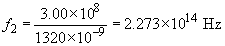The spectral range expressed in frequencies is therefore: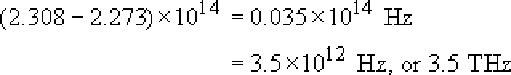Notice that you have to be careful with the accuracy of the intermediate steps in this calculation because it involves subtracting two large and similar numbers.

For small spectral widths there is an approximation formula to convert between spectral width measured in wavelength (Δλ) and spectral width measured in frequency (Δf):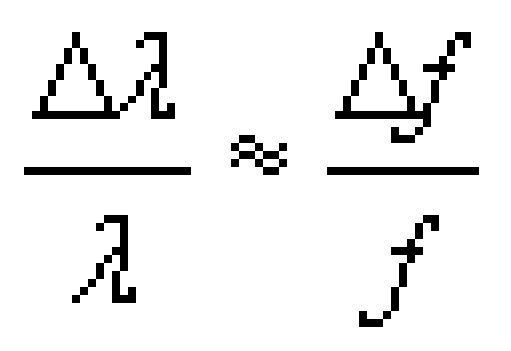where λ and f are taken at the mid-point of the range. This approximation is valid provided the fractions are small – less than about 1/10, for which we require Δλ < λ/10. In fact, because the approximation avoids the need to subtract two large numbers, it can be a better way of doing the calculation.

Often we may know λ but not f, so we can use f = c/λ to get: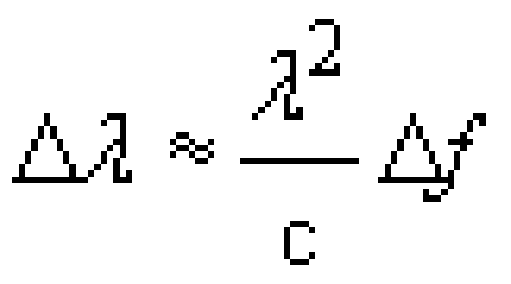and therefore: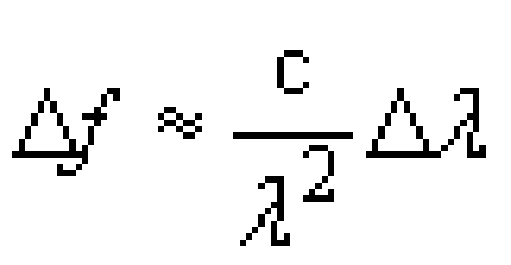Using the example of the LED again, taking λ in the middle of the range at 1310 nm gives: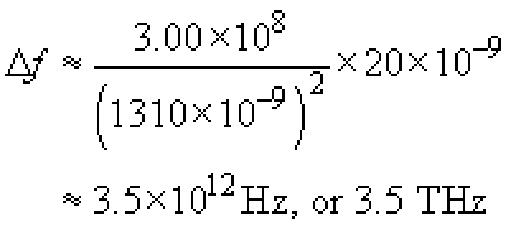## SAQ 1

Suppose an LED has a spectrum that extends from 1540 nm to 1560 nm. Calculate:

1. its spectral width in nm

2. its centre frequency in Hz

3. its spectral width in Hz.

1. Its spectral width in nanometres is 1560 – 1540=20 nm.

2. Its central wavelength is 1550 nm, which corresponds to a frequency of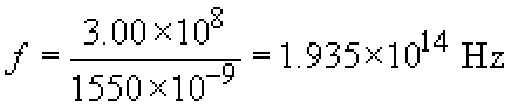3. Its spectrum extends from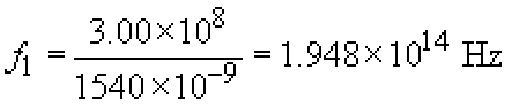to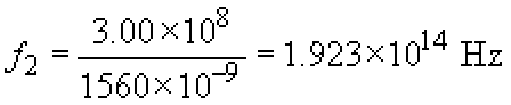which is a spectral width of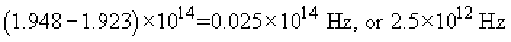Alternatively, this can be calculated using the approximation formula: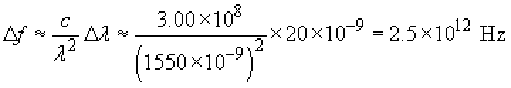Notice that the spectral width of the LED in this SAQ measured in terms of wavelength was the same as that in the example given in Section 1.2, i.e. 20 nm. The spectral width measured in frequency is different, however: 3.5 × 1012 in one case and 2.5 × 1012 here. They are different because the centre wavelength is different.